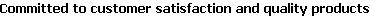Go to content

Main menu:

# Output to File variables

Knowledge Base

The following variables are included in the three text files that are created using the "Output to File" option:

 VARIABLE DEFINITION OVERBANK TABLE OFRESULTS QVA WSEL Water Surface Elevation Yes Yes Yes Q(LOB) Discharge in Left Overbank Yes Q(MCH) Discharge in Main Channel Yes Q(ROB) Discharge in Right Overbank Yes Q(Total XS) Total Cross-section discharge Yes Yes Yes VAve(LOB) Average velocity of Left Overbank flow Yes VAve(MCH) Average velocity of Main Channel flow Yes VAve(ROB) Average velocity of Right Overbank flow Yes VAve(Total XS) Average velocity of Total Cross-section flow Yes Yes Yes Area(LOB) Flow area in Left Overbank Yes Area(MCH) Flow area in Main Channel Yes Area(ROB) Flow area in Right Overbank Yes Area(Total XS) Flow area in Total Cross-section Yes Yes Yes DAve(LOB) Average Depth of Left Overbank flow Yes DAve(MCH) Average Depth of Main Channel flow Yes DAve(ROB) Average Depth of Right Overbank flow Yes DAve(Total XS) Average Depth of Total Cross-section flow Yes Yes DMax(LOB) Maximum Depth of Left Overbank flow Yes DMax(MCH) Maximum Depth of Main Channel flow Yes DMax(ROB) Maximum Depth of Right Overbank flow Yes DMax(Total XS) Maximum Depth of Total Cross-section flow Yes Yes (DAve x V)(LOB) Product of Average Depth and Velocity of Left Overbank flow Yes (DAve x V)(MCH) Product of Average Depth and Velocity of Main Channel flow Yes (DAve x V)(ROB) Product of Average Depth and Velocity of Right Overbank flow Yes (DAve x V)(Total XS) Product of Average Depth and Velocity of Total Cross-section flow Yes Yes (DMax x V)(LOB) Product of Maximum Depth and Velocity of Left Overbank flow Yes (DMax x V)(MCH) Product of Maximum Depth and Velocity of Main Channel flow Yes (DMax x V)(ROB) Product of Maximum Depth and Velocity of Right Overbank flow Yes (DMax x V)(Total XS) Product of Maximum Depth and Velocity of Total Cross-section flow Yes Yes FrNo(LOB) Froude Number of Left Overbank flow Yes FrNo(MCH) Froude Number of Main Channel flow Yes FrNo(ROB) Froude Number of Right Overbank flow Yes FrNo(Total XS) Froude Number of Total Cross-section flow Yes Yes WettedPerim(LOB) Wetted Perimeter of Left Overbank flow Yes WettedPerim(MCH) Wetted Perimeter of Main Channel flow Yes WettedPerim(ROB) Wetted Perimeter of Right Overbank flow Yes WettedPerim(Total XS) Wetted Perimeter of Total Cross-section flow Yes Yes FlowWidth(LOB) Width of Left Overbank flow Yes FlowWidth(MCH) Width of Main Channel flow Yes FlowWidth(ROB) Width of Right Overbank flow Yes FlowWidth(Total XS) Width of Total Cross-section flow Yes Yes Hydr. Radius(LOB) Hydraulic Radius of Left Overbank flow Yes Hydr. Radius(MCH) Hydraulic Radius of Main Channel flow Yes Hydr. Radius(ROB) Hydraulic Radius of Right Overbank flow Yes Hydr. Radius(Total XS) Hydraulic Radius of Total Cross-section flow Yes Yes Composite n(LOB) Composite Manning's n of Left Overbank Yes Composite n(MCH) Composite Manning's n of Main Channel Yes Composite n(ROB) Composite Manning's n of Right Overbank Yes Composite n(Total XS) Composite Manning's n of Total Cross-section Yes Yes